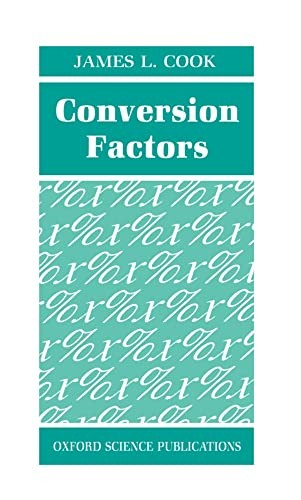Free Shipping on all orders in Australia
Over 7 million books in stock
We aim to be carbon neutral by 2022# Conversion Factors by James L. Cook (Mechanical Engineering Consultant and College Lecturer)

Condition - Very Good
\$15,49
Only 2 left

## Summary

* Useful mathematical and engineering formulae in a convenient and easy to use format This handy reference contains more than 3500 conversion factors which have been calculated from primary conversion factors agreed internationally. All conversion factors are given to the greatest accuracy possible up to ten significant figures.

## Conversion Factors Summary

### Conversion Factors by James L. Cook (Mechanical Engineering Consultant and College Lecturer)

This is a handy reference for students, teachers, and practitioners in engineering, physics, sciences, and technical subjects. More than 3500 conversion factors are given; these have been calculated from primary conversion factors agreed internationally. All conversion factors are given to the greatest accuracy possible up to ten significant figures. The conversion factors are grouped into unit types by areas of science, and range from angular acceleration to volume flow. An appendix of agreed primary conversion factors is given. Also of practical help are appendices of common mathematical and engineering formulae.

## Conversion Factors Reviews

This is an excellent (almost pocket-sized) reference book covering nearly four thousand conversion factors relating to quantities used every day in science, engineering and technology. The layout is exceptionally well organised for ease of reference and the volume also contains a good deal of numerical data. * ASLIB Booklist *

## About James L. Cook (Mechanical Engineering Consultant and College Lecturer)

James L. Cook is a freelance Mechanical Design Engineer

## Table of Contents

ABBREVIATIONS; NOTES; CONVERSION FACTORS; Acceleration (Angular); Acceleration (Linear); Area; Area Flow; Calorific Value (Volume basis); Calorific Value (Volume, British Gas); Chemical (Henry's Law); Circular and Spherical Geometry; Coefficient of Expansion (Volumetric); Concentration (Liquid solution); Density (Mass concentration); Electrical (Charge); Electrical (Conductance); Electrical (Conductivity); Electrical (Current); Electrical (Electric capacitance); Electrical (Electric field); Electrical (Electric potential); Electrical (Inductance); Electrical (Linear charge density); Electrical (Linear current density); Electrical (Magnetic field strength); Electrical (Magnetic flux density); Electrical (Magnetomotive force); Electrical (Permeability); Electrical (Resistance); Electrical (Resistivity); Electrical (Surface charge density); Electrical (Surface current density); Electrical (Volume charge density); Energy (Heat, work, electrical); Enthalpy; Entropy; Force (Weight); Force/Unit Length (Spring rate); Heat Capacity; Heat Flux Density; Heat Transfer Coefficient; Illumination (Luminous flux); Illumination (Luminous incidence); Illumination (Luminous intensity); Linear Measure (Distance or depth); Mass; Mass Flow; Mass Flow Density; Moment of Inertia; Paper; Power; Pressure or Stress; Sound; Specific Energy (Specific heat); Specific Volume; Surface Tension; Temperature; Temperature Difference; Thermal Conductivity; Time; Torque; Velocity (Angular); Velocity (Linear); Viscosity (Absolute, Dynamic); Viscosity (Kinematic); Volume (Capacity); Volume Flow; NUMERICAL DATA; Fluids; Gases; Metals (Strength); Timber; Metals (Thermal); Densities; PHYSICAL CONSTANTS: Atoms and Spectroscopy; Energy, Mass, and Wavelength; Principal Constants; Thermal Constants; MATHEMATICAL FORMULAE; ENGINEERING FORMULAE.

## Additional information

GOR002149660
Conversion Factors by James L. Cook (Mechanical Engineering Consultant and College Lecturer)
Used - Very Good
Hardback
Oxford University Press
1991-06-27
176
0198563493
9780198563495
N/A
Book picture is for illustrative purposes only, actual binding, cover or edition may vary.
This is a used book - there is no escaping the fact it has been read by someone else and it will show signs of wear and previous use. Overall we expect it to be in very good condition, but if you are not entirely satisfied please get in touch with us